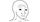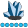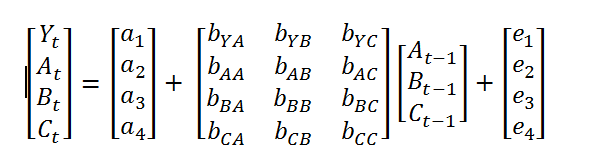somebody
Lapis Lazuli | Level 10

## Vector Autoregression with parameter restriction

Hi all,

I would like to run some Vector Autoregression with parameters restrictions. That is, I dont want to regress the dependent variable (Yt) on its lag but other independent variables are still regressed on their lags. Please see attached file for a matrix illustration. I only want 1 lag

I also would like to obtain the variance-covariance matrix of the error terms. My code so far:

```proc varmax data=input_data;
model Y = A B C/ P=1;
* restrict AR(1, Y, {A B C}) = 0,
XL(0, CPI, {FFR CP}) = 0; *not sure what this restrict option does as why do we set it to equal 0;
run;```1 ACCEPTED SOLUTION

Accepted Solutions

## Re: Vector Autoregression with parameter restriction

Hi,

Based on the description of your model, you can obtain your desired result using the following PROC VARMAX specification:

``````proc varmax data=input_data;
model y a b c / p=1 print=(estimates) ;
restrict ar(1,1,1)=0, ar(1,2,1)=0, ar(1,3,1)=0, ar(1,4,1)=0;
run;``````

Since you want the variables, A, B and C, to also be regressed on their lags, they must appear on the left-hand-side of the equation.

The output generated by PROC VARMAX includes a table labeled "Covariances of Innovations".  This is the variance-covariance matrix of the error terms, which you indicated that you also needed.  If you want to save this variance-covariance matrix as a SAS data set, then you can use an ODS OUTPUT statement to do so.

Following, please find a modification of the above code, which illustrates the ODS OUTPUT statement and also provides a modified version of the RESTRICT statement.  The modified RESTRICT statement imposes the same restriction, but uses a more concise matrix expression.  A PROC PRINT step is added to print the ERROR_COV data set created from the ODS OUTPUT statement.

``````proc varmax data=input_data;
ods output CovInnovation=error_cov;
model y a b c / p=1 print=(estimates) ;
restrict ar(1,{1,2,3,4},1)=0;
run;

proc print data=error_cov;
run;``````

I hope this helps!

DW

2 REPLIES 2

## Re: Vector Autoregression with parameter restriction

Hi,

Based on the description of your model, you can obtain your desired result using the following PROC VARMAX specification:

``````proc varmax data=input_data;
model y a b c / p=1 print=(estimates) ;
restrict ar(1,1,1)=0, ar(1,2,1)=0, ar(1,3,1)=0, ar(1,4,1)=0;
run;``````

Since you want the variables, A, B and C, to also be regressed on their lags, they must appear on the left-hand-side of the equation.

The output generated by PROC VARMAX includes a table labeled "Covariances of Innovations".  This is the variance-covariance matrix of the error terms, which you indicated that you also needed.  If you want to save this variance-covariance matrix as a SAS data set, then you can use an ODS OUTPUT statement to do so.

Following, please find a modification of the above code, which illustrates the ODS OUTPUT statement and also provides a modified version of the RESTRICT statement.  The modified RESTRICT statement imposes the same restriction, but uses a more concise matrix expression.  A PROC PRINT step is added to print the ERROR_COV data set created from the ODS OUTPUT statement.

``````proc varmax data=input_data;
ods output CovInnovation=error_cov;
model y a b c / p=1 print=(estimates) ;
restrict ar(1,{1,2,3,4},1)=0;
run;

proc print data=error_cov;
run;``````

I hope this helps!

DWsomebody
Lapis Lazuli | Level 10

## Re: Vector Autoregression with parameter restriction

Thanks, this works fine.

Discussion stats
• 2 replies
• 776 views
• 1 like
• 2 in conversation